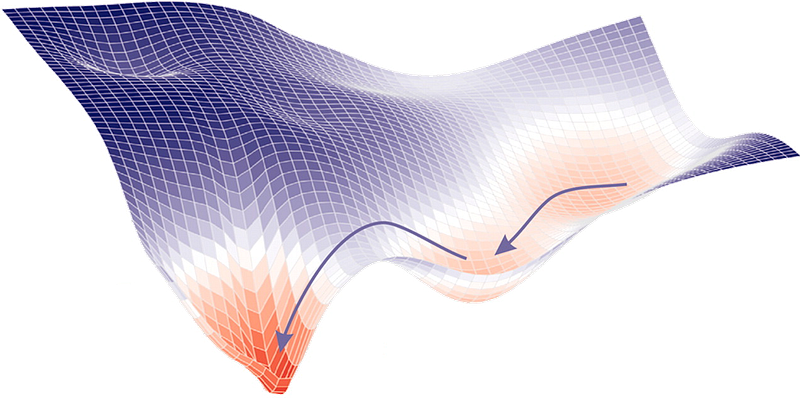# Gradient Descent Optimization & ChallengesGradient descent is an optimization algorithm used to minimize some function by iteratively moving in the direction of steepest descent as defined by the negative of the gradient. In machine learning, we use gradient descent to update the parameters of our model. Parameters refer to coefficients in Linear Regression and weights in neural networks. Gradient Descent | ML Cheatsheet

Gradient Descent is the most common optimization algorithm in machine learning and deep learning. It is a first-order optimization algorithm. This means it only takes into account the first derivative when performing the updates on the parameters. On each iteration, we update the parameters in the opposite direction of the gradient of the objective function J(w) w.r.t the parameters where the gradient gives the direction of the steepest ascent. Gradient Descent Algorithm and Its Variants | Imad Dabbura - Towards Data Science

Nonlinear Regression algorithms, which fit curves that are not linear in their parameters to data, are a little more complicated, because, unlike linear Regression problems, they can’t be solved with a deterministic method. Instead, the nonlinear Regression algorithms implement some kind of iterative minimization process, often some variation on the method of steepest descent. Steepest descent basically computes the squared error and its gradient at the current parameter values, picks a step size (aka learning rate), follows the direction of the gradient “down the hill,” and then recomputes the squared error and its gradient at the new parameter values. Eventually, with luck, the process converges. The variants on steepest descent try to improve the convergence properties. Machine learning algorithms are even less straightforward than nonlinear Regression, partly because machine learning dispenses with the constraint of fitting to a specific mathematical function, such as a polynomial. There are two major categories of problems that are often solved by machine learning: Regression and classification. Regression is for numeric data (e.g. What is the likely income for someone with a given address and profession?) and classification is for non-numeric data (e.g. Will the applicant default on this loan?). Machine learning algorithms explained | Martin Heller - InfoWorld